Home > ACC7 > Chapter cc27 > Lesson cc27.1.5 > Problem7-60

7-60.
1. Write the inequality represented by each graph. Homework Help ✎

1.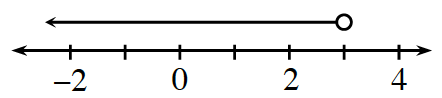2.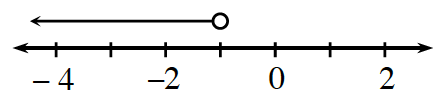3.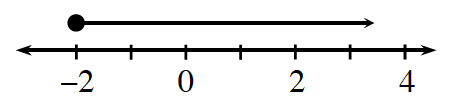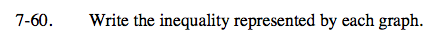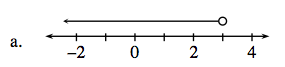Since the arrow is pointing to the left, that means that all those values are less than the selected point.

x < 3

Therefore, any value to the left of 3 is less than 3. Since the circle above the selected point is open, the point itself is not included and you need to use either < or >.See part (a).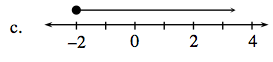Notice that this time the circle above the selected point is shaded in, so the point itself is included. That means you will use either ≤ or ≥. Since the arrow is pointing to the right, that means that all those values are greater than the selected point.